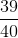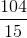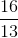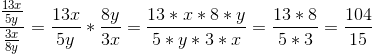## Example Questions

### Example Question #8 : Operations With Fractions

Simplify: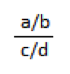a/b/c/d

ac/bd

a2/c2

It is already in simplest terms

Explanation:

Division is the same as multiplying by the reciprocal.  Thus, a/b ÷ c/d = a/b x d/c = ad/bc

### Example Question #1491 : Sat Mathematics

If p is a positive integer, and 4 is the remainder when p-8 is divided by 5, which of the following could be the value of p?

19

20

17

18

17

Explanation:

Remember that if x has a remainder of 4 when divided by 5, x minus 4 must be divisible by 5. We are therefore looking for a number p such that p - 8 - 4 is divisible by 5. The only answer choice that fits this description is 17.

### Example Question #10 : Operations With Fractions

If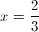and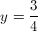, then what is the value of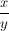?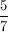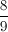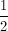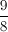Explanation:

Dividing by a number (in this case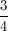) is equivalent to multiplying by its reciprocal (in this case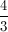).  Therefore: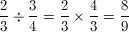### Example Question #11 : Operations With Fractions

Evaluate the following: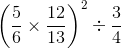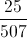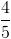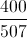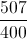Explanation:First we will evaluate the terms in the parentheses: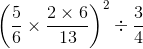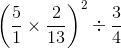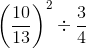Next, we will square the first fraction: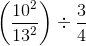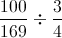We can evaluate the division as such: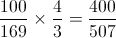### Example Question #1 : How To Divide Fractions

Evaluate the expression: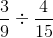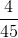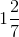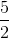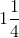Explanation:

When dividing fractions, you invert the second term and multiply the numbers.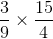You can reduce the numbers that are diagonal from each other to make the numbers smaller and easier to multiply.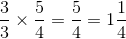### Example Question #2 : How To Divide Fractions

Simplify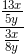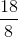Fractions with variables cannot be divided